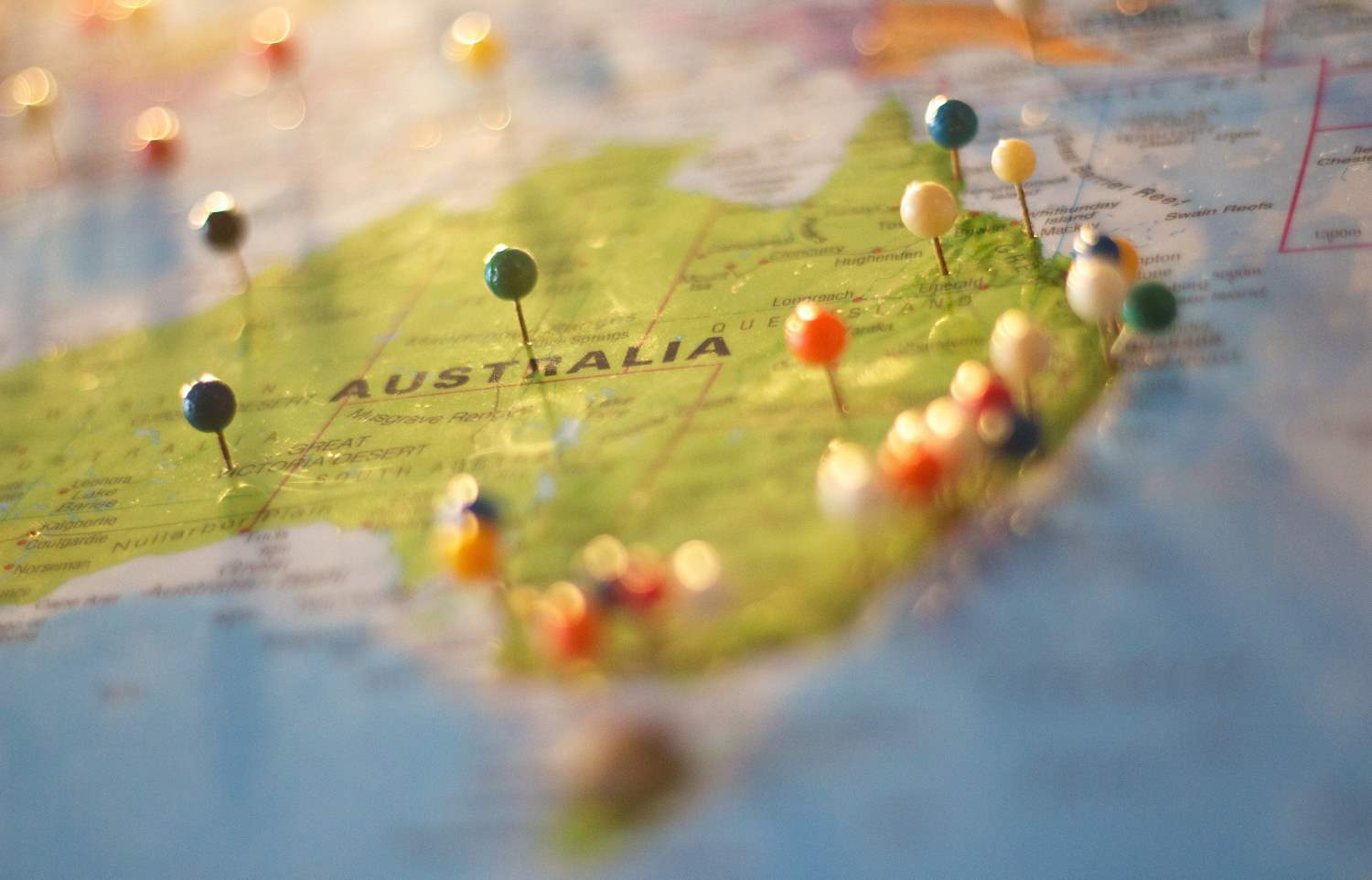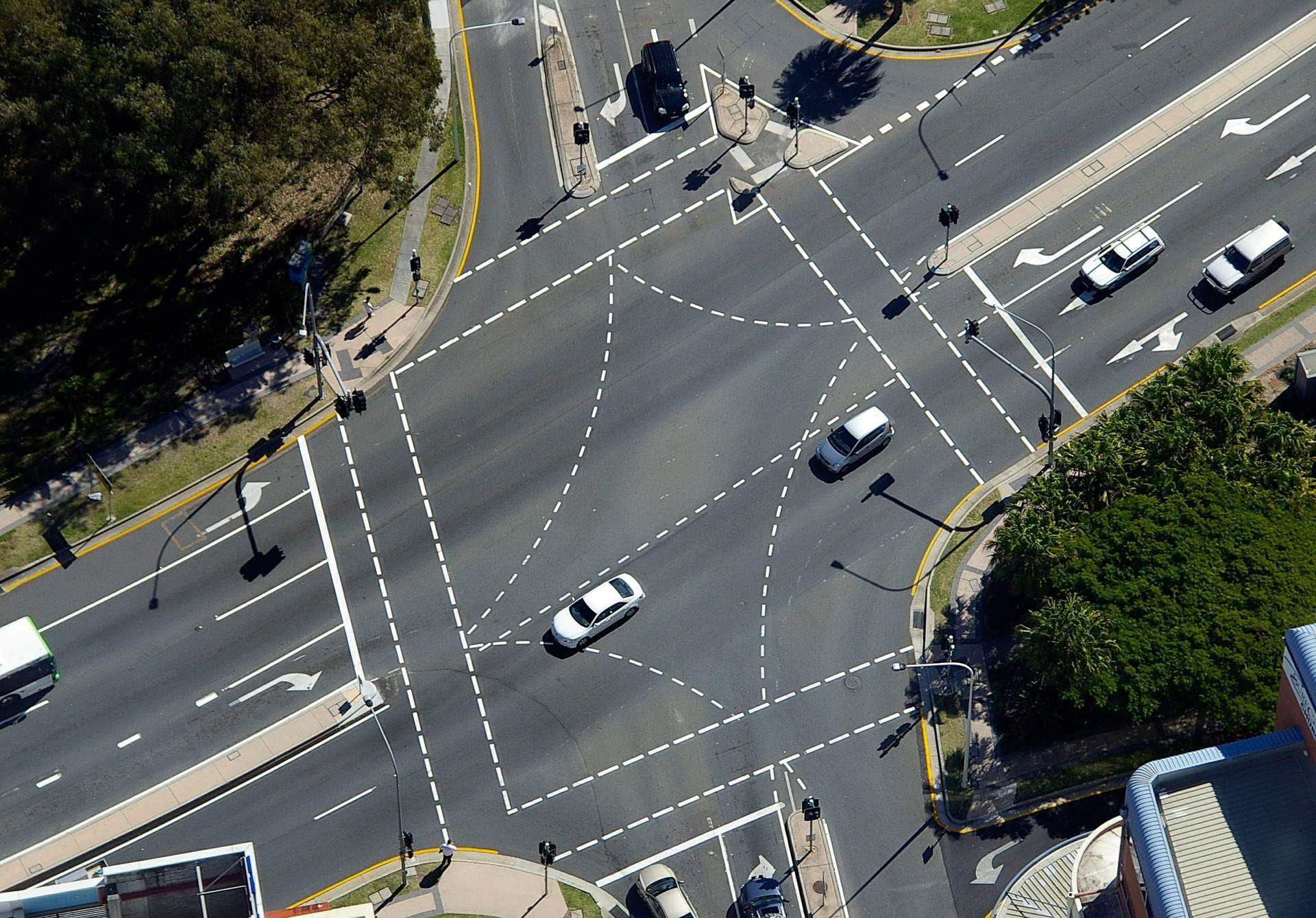# How do you work out speed vs time vs distance?

There are simple equations you can use if you want to estimate how long it’ll take you to get somewhere.

Distance = Speed * Time

You can make any of these variables (or ‘terms’) the subject of the equation, therefore:

Speed = Distance / Time

Time = Distance / Speed

Nice and simple – now you need to make sure your numbers match the result you want. For example, you can’t plug in a time in minutes and a distance in miles and expect it to come back with a speed in kilometres per hour. We need to do some quick conversions of your numbers.

Convert miles into kilometres: Multiply by 1.60934

Convert kilometres into miles: Divide by 1.60934

For example, 10 miles is 16.09km.

We also need to convert time into a decimal figure. For example, how do you convert 3 hours 45 minutes and 16 seconds into a decimal figure?

First, take the seconds and divide it by 60 (there are 60 seconds in a minute)

16/60 = 0.267

Add that to the 45 minutes = 45.267. I.e. 45 minutes 16 seconds is 45.27 minutes. But now we need to find out what 45.27 minutes is in relation to an hour, so we need to divide it by 60 (there are 60 minutes in an hour)

45.27/60 = 0.754

Add that to the number of hours (3) and you get 3.754 hours. Now we can deal with equations in miles or kilometres per hour. If you leave the figure in minutes you will be dealing in miles or kilometres per minute.

Bear in mind that we rounded the figures, i.e. where it was 0.267 it was actually 0.2666666666 (recurring) and this will create a rounding error when we calculated it back out in reverse. To do this in reverse (i.e. to make 3.754 into hours, minutes and seconds), first take the fractional part (0.754) and multiply it by 60.

0.754 * 60 = 45.24

We’ve ended up with .24 of a minute which we need to convert to seconds.

0.24 * 60 = 14.4 seconds, or 14 seconds if we want to round it down.

Therefore we have 3 hours, 45 minutes and 14 seconds: we’re back to where we were but with a two-second rounding error (remember the original figure was 3 hours 45 minutes and 16 seconds).

Now, onto calculating speed, distance and time.

## How far will a car travel in a certain amount of time?

You’ll need to know the distance and the speed to calculate this. Note that we are simply dealing with the amount of ground covered, not necessarily how far away from a certain point the car will be because if the path the car takes is curved, the distance it has travelled will be longer than the distance it is from the start. Anyway, let’s assume that we’re travelling at 72km/h for 4 hours 12 minutes and 29 seconds.

Convert 4 hours 12 minutes and 29 seconds into decimal time = 4.21 hours.

Distance = speed * time, therefore 72km/h * 4.21 hours = 303km when rounded to the nearest kilometre.

## How fast is a car travelling if it travels 14 kilometres in 13 minutes?

The equation is speed = distance / time. We have a distance (14) and a time (13), but we need to convert the time to decimal time.

13/60 = 0.2166666666 hours.

Divide 14/0.217 = 64.62km/h

## If a car is travelling at a certain speed, how long will it take to do a certain distance?

Let’s say that you are travelling at 103km/h and you need to drive 198km.

Time = distance / speed = 198km  / 103km/h = 1.922 hours.

Let’s convert the .922 into minutes by multiplying it by 60 and we have 55.34 minutes.

Let’s convert the 0.34 minutes into seconds by multiplying it by 60 and we get 20.4 seconds, or 20 seconds if we round it down.

Therefore, it will take 1 hour 55 minutes and 20 seconds to cover 198km at 103km/h.Darren is an expert on driving and transport, and is a member of the Institute of Advanced Motorists

Tagged with: